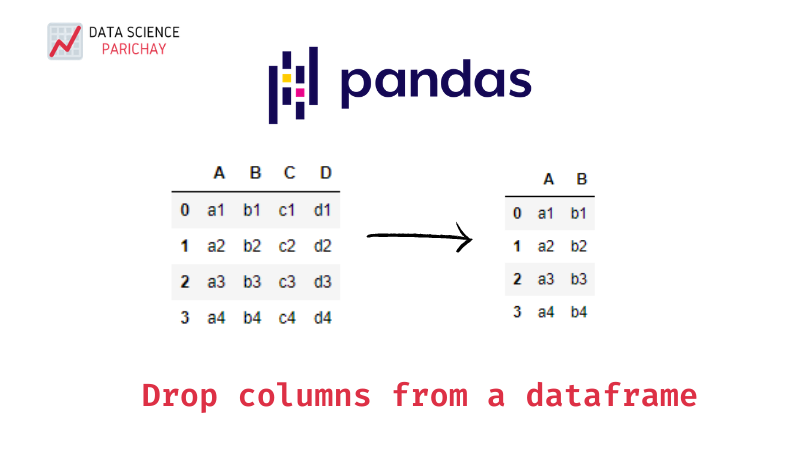# Pandas – Drop one or more Columns from a Dataframe

Pandas dataframes are quite powerful for manipulating data. Often while working with data particularly during EDA (Exploratory Data Analysis) and data preprocessing, you may require to remove one or more columns. In this tutorial, we’ll cover how to drop one or more columns from a pandas dataframe with some examples.

You can use the pandas dataframe `drop()` function with axis set to 1 to remove one or more columns from a dataframe. The following is the syntax:

``df.drop(cols_to_drop, axis=1)``

Here, cols_to_drop the is index or column labels to drop, if more than one columns are to be dropped it should be a list. The axis represents the axis to remove the labels from, it defaults to 0 but if you want to drop columns pass the axis as 1 (i.e. `0` for rows and `1` for columns).

Also note that the `drop()` function does not modify the dataframe in-place by default. It returns a copy of the dataframe with the labels dropped. If you want to modify the dataframe in-place pass the argument `inplace=True` to the function.

To drop columns by name simply pass the column name (if you want to drop a single column) or the list of columns (if you want to drop multiple columns) to the drop function. See the examples below:

Example 1: Drop a single column by name

``````import pandas as pd

# create a sample dataframe
data = {
'A': ['a1', 'a2', 'a3'],
'B': ['b1', 'b2', 'b3'],
'C': ['c1', 'c2', 'c3'],
'D': ['d1', 'd2', 'd3']
}

df = pd.DataFrame(data)

# print the dataframe
print("Original Dataframe:\n")
print(df)

# remove column C
df = df.drop('C', axis=1)

print("\nAfter dropping C:\n")
print(df)``````

Output:

``````Original Dataframe:

A   B   C   D
0  a1  b1  c1  d1
1  a2  b2  c2  d2
2  a3  b3  c3  d3

After dropping C:

A   B   D
0  a1  b1  d1
1  a2  b2  d2
2  a3  b3  d3``````

In the above example, a sample dataframe `df` is created with four columns `A`, `B`, `C`, and `D`. Then, the column `C` is dropped using the drop() function. Notice that since we had to drop just a single column we didn’t need to pass a list.

📚 Data Science Programs By Skill Level

Introductory

Intermediate ⭐⭐⭐

🔎 Find Data Science Programs 👨‍💻 111,889 already enrolled

Disclaimer: Data Science Parichay is reader supported. When you purchase a course through a link on this site, we may earn a small commission at no additional cost to you. Earned commissions help support this website and its team of writers.

Example 2: Drop multiple columns by name

``````import pandas as pd

# create a sample dataframe
data = {
'A': ['a1', 'a2', 'a3'],
'B': ['b1', 'b2', 'b3'],
'C': ['c1', 'c2', 'c3'],
'D': ['d1', 'd2', 'd3']
}

df = pd.DataFrame(data)

# print the dataframe
print("Original Dataframe:\n")
print(df)

# remove columns C and D
df = df.drop(['C', 'D'], axis=1)

print("\nAfter dropping columns C and D:\n")
print(df)``````

Output:

``````Original Dataframe:

A   B   C   D
0  a1  b1  c1  d1
1  a2  b2  c2  d2
2  a3  b3  c3  d3

After dropping columns C and D:

A   B
0  a1  b1
1  a2  b2
2  a3  b3
``````

In the above example, the columns `C` and `D` are dropped from the dataframe `df`. Note that we had to provide the list of column names to drop since we were dropping multiple columns together.

To drop columns by column number, pass `df.columns[i]` to the drop() function where `i` is the column index of the column you want to drop. To drop multiple columns by their indices pass `df.columns[[i, j, k]]` where `i`, `j`, `k` are the column indices of the columns you want to drop.

Example 1: Drop a single column by index

``````import pandas as pd

# create a sample dataframe
data = {
'A': ['a1', 'a2', 'a3'],
'B': ['b1', 'b2', 'b3'],
'C': ['c1', 'c2', 'c3'],
'D': ['d1', 'd2', 'd3']
}

df = pd.DataFrame(data)

# print the dataframe
print("Original Dataframe:\n")
print(df)

# remove column
df = df.drop(df.columns, axis=1)

print("\nAfter dropping C:\n")
print(df)``````

Output:

``````Original Dataframe:

A   B   C   D
0  a1  b1  c1  d1
1  a2  b2  c2  d2
2  a3  b3  c3  d3

After dropping C:

A   B   D
0  a1  b1  d1
1  a2  b2  d2
2  a3  b3  d3
``````

In the above example, the column `C` is dropped using its index `2` from the dataframe `df`.

Example 2: Drop multiple columns with their index

``````import pandas as pd

# create a sample dataframe
data = {
'A': ['a1', 'a2', 'a3'],
'B': ['b1', 'b2', 'b3'],
'C': ['c1', 'c2', 'c3'],
'D': ['d1', 'd2', 'd3']
}

df = pd.DataFrame(data)

# print the dataframe
print("Original Dataframe:\n")
print(df)

# remove columns C and D
df = df.drop(df.columns[[2, 3]], axis=1)

print("\nAfter dropping columns C and D:\n")
print(df)``````

Output:

``````Original Dataframe:

A   B   C   D
0  a1  b1  c1  d1
1  a2  b2  c2  d2
2  a3  b3  c3  d3

After dropping columns C and D:

A   B
0  a1  b1
1  a2  b2
2  a3  b3
``````

In the above example, columns with index `2` and `3` are dropped from the dataframe `df`.

Behind the scenes, `df.columns` actually gives the name of the column for the given index:

``df.columns``

Output:

``'A'``

For more on the `drop()` function and its syntax refer to its documentation.

With this, we come to the end of this tutorial. The code examples and results presented in this tutorial have been implemented in a Jupyter Notebook with a python (version 3.8.3) kernel having pandas version 1.0.5

•